Search

Solving Polynomial Inequalities: Examples (page 2 of 2)• Find the solution:
(x  5)2(x  1)(x + 3) < 0
• For this polynomial, I'm looking for where the graph is below the axis. And since this is an "or equal to" inequality, I'll be including the interval endpoints, the zeroes, in my solution.

The zeroes are at x = 5 (twice), x = 1, and x = 3, so my intervals are (infinity, 3), (3, 1), (1, 5), and (5, +infinity). If I went to the trouble of multiplying the factors together, I'd get a positive quartic (degree-4) polynomial whose graph crosses the x-axis at x = 3 and x = 1, but only touches (and then turns back the way it came) at x = 5, so the graph would look something like this:So my solution will include the one interval where the polynomial is below the axis, (3, 1), along with that interval's endpoints. In addition, I'll also have the one other point (x = 5) where the polynomial is equal to zero. But supposing I weren't so familiar with the polynomial's shape and behavior, I could still find the answer with a factor table. I'll make a table of the intervals and the factors: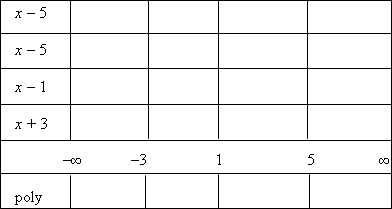Now I'll insert the factors' signs on the various intervals: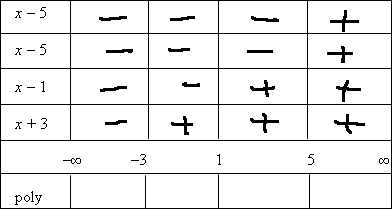...and now I'll multiply the signs on each interval to find the sign of the polynomial on that interval: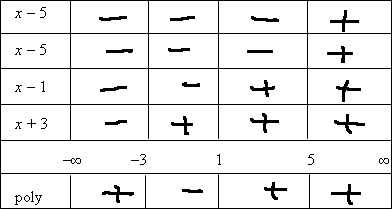Then the solution, including the "or equal to" part, is:

[3, 1] and {5}

Since this was an "or equal to" inequality, the endpoints had to be included, since they are where the polynomial equalled zero. That's why I had to throw "x = 5" into the solution.

Notice the formatting of the "interval" containing only the one point, x = 5. This is called a "singleton" set, because it contains only one point. Use curly braces, as I did above, to indicate just the one point, or else use inequality notation (if you're allowed) to write the solution as "3 < x < 1 and x = 5". Always be careful with "or equal to" inequalities, and remember to check the endpoints; you may have a singleton set that is part of the solution or you might find that two intervals are actually joined into one by a shared endpoint.

• Find the solution: (x2 + 2)(x + 5)3(4  2x)(x2)(x  6) < 0
• The zeroes are at x = 5, x = 2, x = 0, and x = 6. But to find the signs on the factors, I'll need to be a little more thorough, because I've got a "backwards" factor (the "4  2x") and a zero-free factor (the "x2 + 2").   Copyright © Elizabeth Stapel 2005-2011 All Rights Reserved

x2 + 2 > 0 always

x + 5 > 0 for x > 5

4  2x = 2(x  2), giving me two factors: 2 and x  2

2 > 0 never and x  2 > 0 for x > 2

x2 = (x)(x): x > 0 for x > 0

x  6 > 0 for x > 6

Here's my factor table with the signs inserted: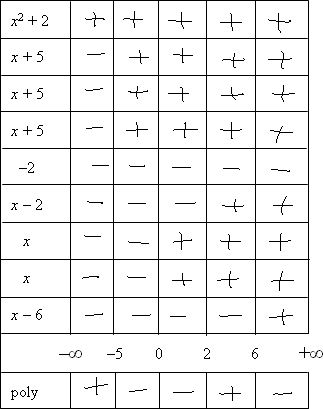(Note the doubled and tripled factors, corresponding to the powers on those factors. Don't forget to take factors' "multiplicities" into account.)

Then the solution intervals are (5, 0), (0, 2), and (6, infinity). But this is an "or equal to" inequality, so I also have to include the endpoints. That is, the points 5, 0, 2, and 6 are also part of the solution. By adding 0 to the solution, I will join the two intervals (5, 0) and (0, 2). So I'll write these two as one, turning "[5, 0] and [0, 2]" into "[5, 2]". Then the whole solution is: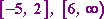Note: "Infinity" isn't a number, so it's never "included" as an interval endpoint. Never draw a square bracket at "infinity".

<< Previous  Top  |  1 | 2  |  Return to Index

 Cite this article as: Stapel, Elizabeth. "Solving Polynomial Inequalities: Examples." Purplemath. Available from     https://www.purplemath.com/modules/ineqpoly2.htm. Accessed [Date] [Month] 2016

Study Skills Survey

Tutoring from Purplemath
Find a local math tutor

 Copyright © 2021  Elizabeth Stapel   |   About   |   Terms of Use   |   Linking   |   Site Licensing Contact Us